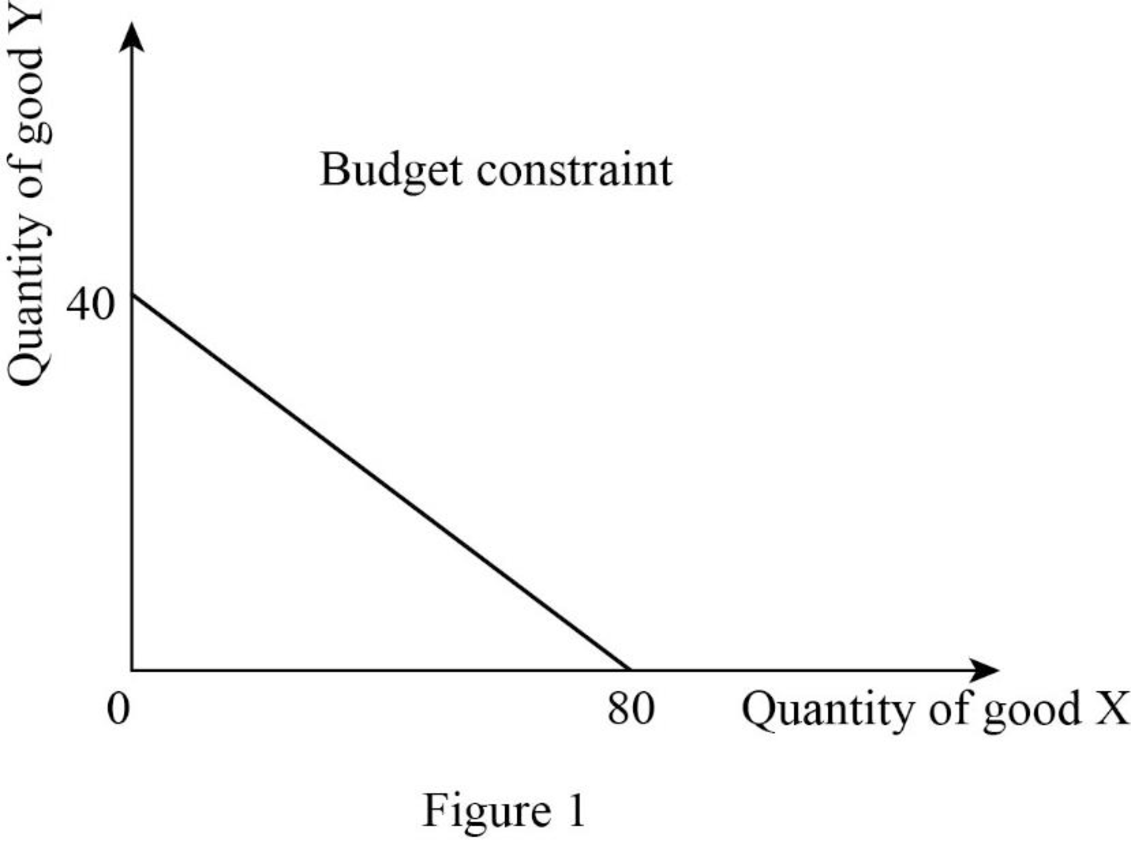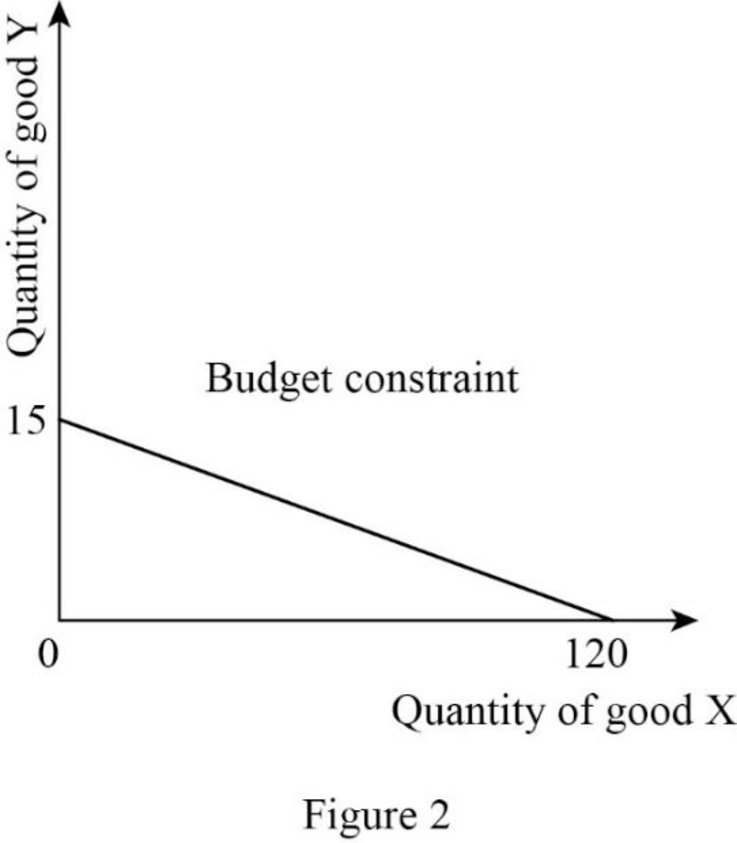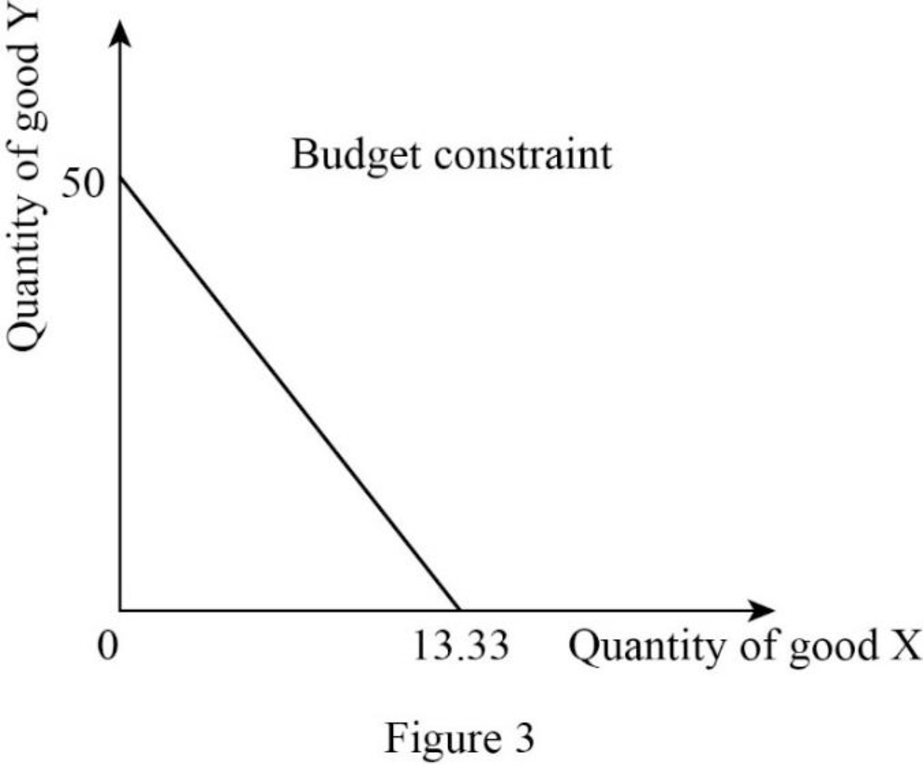# Diagram the following budget constraints: a. Income = $4,000; P X =$50; P Y = $100 b. Income =$3,000; P X = $25; P Y =$200 c. Income = $2,000; P X =$40; P Y = $150BuyFind ### Microeconomics 13th Edition Roger A. Arnold Publisher: Cengage Learning ISBN: 9781337617406BuyFind ### Microeconomics 13th Edition Roger A. Arnold Publisher: Cengage Learning ISBN: 9781337617406 #### Solutions Chapter Section Chapter C, Problem 1QP Textbook Problem ## Diagram the following budget constraints: a. Income =$4,000; PX = $50; PY =$100 b. Income = $3,000; PX =$25; PY = $200 c. Income =$2,000; PX = $40; PY =$150

Expert Solution

(a)

To determine

The budget constraint.

### Explanation of Solution

Since the income is $4,000 and price of good X is$50, the quantity of good X can be calculated as follows:

Quantity of good X=IncomePrice of good X=$4,000$50=80

Thus, the consumer will consume 80X.

Since the income is $4,000 and price of good Y is$100, the quantity of good Y can be calculated as follows:

Quantity of good Y=IncomePrice of good Y=$4,000$100=40

Thus, the consumer will consume 40Y.

Now, the budget constraint can be represented as follows:In Figure 1, the vertical axis measures the quantity of good Y and the horizontal axis measures the quantity of good X.

Economics Concept Introduction

Budget constraints: Budget constraint refers to the possible combination of goods and services that a consumer can purchase at a given price level with the entire income.

Expert Solution

(b)

To determine

The budget constraint.

### Explanation of Solution

Since the income is $3,000 and price of good X is$25, the quantity of good X can be calculated as follows:

Quantity of good X=IncomePrice of good X=$3,000$25=120

Thus, the consumer will consume 120X.

Since the income is $3,000 and price of good Y is$200, the quantity of good Y can be calculated as follows:

Quantity of good Y=IncomePrice of good Y=$3,000$200=15

Thus, the consumer will consume 15Y.

Now, the budget constraint can be represented as follows:In Figure 2, the vertical axis measures the quantity of good Y and the horizontal axis measures the quantity of good X.

Economics Concept Introduction

Budget constraints: Budget constraint refers to the possible combination of goods and services that a consumer can purchase at a given price level with the entire income.

Expert Solution

(c)

To determine

The budget constraint.

### Explanation of Solution

Since the income is $2,000 and price of good X is$40, the quantity of good X can be calculated as follows:

Quantity of good X=IncomePrice of good X=$2,000$40=50

Thus, the consumer will consume 50X.

Since the income is $2,000 and price of good Y is$150, the quantity of good Y can be calculated as follows:

Quantity of good Y=IncomePrice of good Y=$2,000$150=13.33

Thus, the consumer will consume 13.33Y.

Now, the budget constraint can be represented as follows:In Figure 3, the vertical axis measures the quantity of good Y and the horizontal axis measures the quantity of good X.

Economics Concept Introduction

Budget constraints: Budget constraint refers to the possible combination of goods and services that a consumer can purchase at a given price level with the entire income.

### Want to see more full solutions like this?

Subscribe now to access step-by-step solutions to millions of textbook problems written by subject matter experts!

Get Solutions

### Want to see more full solutions like this?

Subscribe now to access step-by-step solutions to millions of textbook problems written by subject matter experts!

Get Solutions

Find more solutions based on key concepts
Show solutions
What are cash equivalents?

College Accounting, Chapters 1-27 (New in Accounting from Heintz and Parry)

How does nonstore retailing occur?

Foundations of Business (MindTap Course List)

How are managements actions incorporated in EVA and MVA? How are EVA and MVA interconnected?

Fundamentals of Financial Management, Concise Edition (with Thomson ONE - Business School Edition, 1 term (6 months) Printed Access Card) (MindTap Course List)

Is savings harmful or beneficial to the economy? Contrast the two views on this issue.

Economics: Private and Public Choice (MindTap Course List)

3. Why do corporations issue stock?

Cornerstones of Financial Accounting